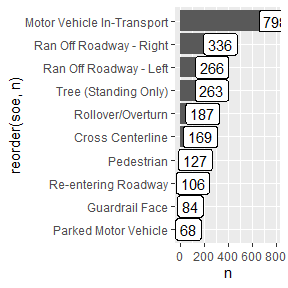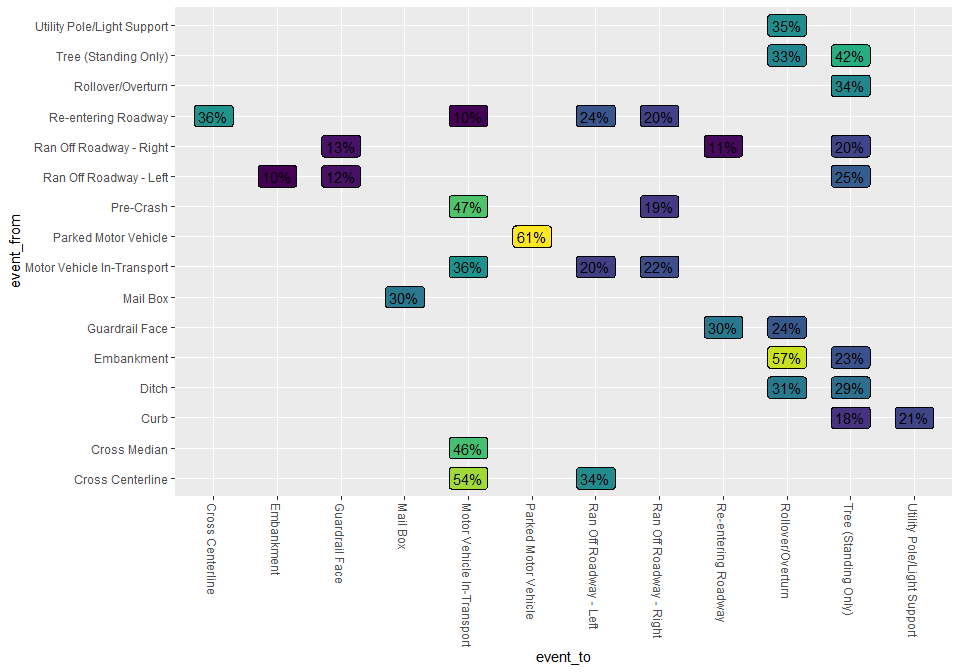# Crash Sequences

This vignette explores the Vsoe (Vehicle Sequence of Events) data to visualize crash sequence patterns.

Vsoe is one of three event-based data files, the others being Cevent and Vevent. According to the data dictionary, Vevent “has the same data elements as the Cevent data file” plus “a data element that records the sequential event number for each vehicle,” and the Vsoe file “has a subset of the data elements contained in the Vevent data file (it is a simplified Vevent data file)” (p. 16). rfars therefore omits Cevent and Vevent.

Below we get one year of data for one state, Virginia.

myFARS <- get_fars(years = 2020, states = "VA")

The Vsoe data is stored in the events tibble of the object returned by get_fars(). Here we see the top 10 individual events:

myFARS$events %>% group_by(soe) %>% summarize(n=n()) %>% arrange(desc(n)) %>% slice(1:10) %>% ggplot(aes(x=n, y=reorder(soe, n), label=scales::comma(n))) + geom_col() + geom_label()We can also see the top 10 most common sequences: myFARS$events %>%
select(-aoi) %>%
pivot_wider(names_from = "veventnum", values_from = "soe", values_fill = "x",
names_prefix = "event") %>%
select(starts_with("event")) %>%
group_by_all() %>%
summarize(n=n(), .groups = "drop") %>%
arrange(desc(n)) %>%
slice(1:10) %>%
select(event1, event2, n)
#> # A tibble: 10 × 3
#>    event1                     event2                         n
#>    <chr>                      <chr>                      <int>
#>  1 Motor Vehicle In-Transport x                            421
#>  2 Pedestrian                 x                             97
#>  3 Cross Centerline           Motor Vehicle In-Transport    52
#>  4 Motor Vehicle In-Transport Motor Vehicle In-Transport    42
#>  5 Parked Motor Vehicle       x                             34
#>  6 Ran Off Roadway - Right    Tree (Standing Only)          25
#>  7 Ran Off Roadway - Left     Tree (Standing Only)          24
#>  8 Motor Vehicle In-Transport Rollover/Overturn             10
#>  9 Motor Vehicle In-Transport Ran Off Roadway - Left         9
#> 10 Motor Vehicle In-Transport Ran Off Roadway - Right        9

Below we consider all state transitions - the transition from one event to the next in the sequence. For example, the sequence A-B-C-D has three transitions: A to B, B to C, and C to D. The graph below shows a subset of the more common transitions in the crash sequences. It is interpreted as follows: the event on the y-axis was followed by the event on the x-axis in the percentage of sequences shown at the intersection of the two events. For example, vehicles went from ‘re-entering roadway’ to ‘cross centerline’ in 36% of sequences. Note that we have added a state labelled ‘Pre-Crash’ to help account for sequences with just one event.

myFARS\$events %>%
group_by(year, state, st_case, veh_no) %>%
dplyr::rename(event_to = soe) %>%
mutate(event_from = data.table::shift(event_to, fill = "Pre-Crash")) %>%
select(event_from, event_to) %>%
group_by(event_from, event_to) %>% summarize(n=n()) %>%
group_by(event_from) %>% mutate(n_from = sum(n)) %>%
mutate(n_pct = n/n_from) %>%
filter(n_pct>.1, n>5) %>%
mutate(event_from = stringr::str_wrap(event_from, 40),
event_to = stringr::str_wrap(event_to, 40)) %>%

ggplot(aes(y=event_from, x=event_to, fill=n_pct, label=scales::percent(n_pct, accuracy = 1))) +
viridis::scale_fill_viridis() +
geom_label() +
theme(
axis.text.x.bottom = element_text(angle=270, hjust = 0, vjust=.5),
legend.position = "none"
)
#> Adding missing grouping variables: year, state, st_case, veh_no
#> summarise() has grouped output by 'event_from'. You can override using the
#> .groups argument.